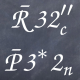# Solution of ODE expressed with hyperbolic trigonometric functionWith Sage 7.3, consider

t=var('t')
v=function('v')(t)
m, g, h = var('m g h')
assume(m > 0)
assume(g > 0)
assume(h > 0)
sol = desolve(m*diff(v,t) == m*g - h*v**2, v, ivar=t)
show(sol)
sol.solve(v)


The outcomes are

$$\newcommand{\Bold}{\mathbf{#1}}-\frac{m \log\left(\frac{h v\left(t\right) - \sqrt{g h m}}{h v\left(t\right) + \sqrt{g h m}}\right)}{2 \, \sqrt{g h m}} = C + t$$

$$\newcommand{\Bold}{\mathbf{#1}}\left[v\left(t\right) = \frac{\sqrt{g h m} {\left(e^{\left(\frac{2 \, \sqrt{g h m} C}{m} + \frac{2 \, \sqrt{g h m} t}{m}\right)} + 1\right)}}{h {\left(e^{\left(\frac{2 \, \sqrt{g h m} C}{m} + \frac{2 \, \sqrt{g h m} t}{m}\right)} - 1\right)}}\right]$$

Nevermind desolve came with an implicit solution I had to further solve by hand but then solve missed that the last expression for v(t) is a mere hyperbolic tangent. How would I get the solution in its simpler form?

$$v(t) = \sqrt{\frac{gm}{h}}\ \text{tanh}\left(\sqrt{\frac{gh}{m}}(t + m C)\right)$$

Thanks for any hint!

edit retag close merge delete

It doesn't help for the hyperbolic ones, but maxima does have some routines to go back and forth between exponential and trigonometric expressions:

sage: tan(x)._maxima_().exponentialize().sage()
(-I*e^(I*x) + I*e^(-I*x))/(e^(I*x) + e^(-I*x))
sage: tan(x)._maxima_().exponentialize().demoivre().sage()
sin(x)/cos(x)


I don't think these routines are directly exposed in sage and "demoivre" doesn't know about hyperbolic routines.

In general, I would expect computer algebra system would give preference to exponential expressions, because it's easy to define a "normal form" for them, whereas it's not so clear what that should mean for hyperbolic/trigonometric functions in general: there's too much redundancy.

Thanks a lot for those hints: I had no idea I could get access to Maxima in such a way. As for my problem, from a human point of view, it is natural to want the "shorter" answer. I have just checked that Mathematica does actually produce the formula with tanh. Besides, the math behind exponentialize and demoivre must have an equivalent in hyperbolic trigonometry.